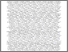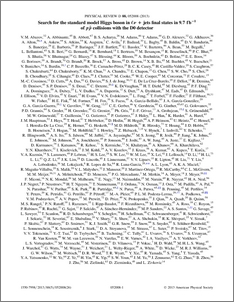# Search for the standard model Higgs boson in $\bm{\ellν}$+jets final states in 9.7~fb$\bm{^{-1}}$ of $\bm{p\bar{p}}$ collisions with the D0 detector

Collaboration, D0 and Bertram, Iain and Borissov, Guennadi and Fox, Harald and Ratoff, Peter (2013) Search for the standard model Higgs boson in $\bm{\ellν}$+jets final states in 9.7~fb$\bm{^{-1}}$ of $\bm{p\bar{p}}$ collisions with the D0 detector. Physical Review D, 88 (5). ISSN 1550-7998Preview
PDF (PhysRevD.88.052008)
PhysRevD.88.052008.pdf - Published Version

## Abstract

We present, in detail, a search for the standard model Higgs boson, $H$, in final states with a charged lepton (electron or muon), missing energy, and two or more jets in data corresponding to 9.7 fb$^{-1}$ of integrated luminosity collected at a center of mass energy of $\sqrt{s}$ = 1.96 TeV with the D0 detector at the Fermilab Tevatron $p\bar{p}$ Collider. The search uses $b$-jet identification to categorize events for improved signal versus background separation and is sensitive to associated production of the $H$ with a $W$ boson, $WH\to\ell\nu b\bar{b}$; gluon fusion with the Higgs decaying to $W$ boson pairs, $H\to WW\to\ell\nu jj$; and associated production with a vector boson where the Higgs decays to $W$ boson pairs, $VH\to VWW \to \ell\nu jjjj$ production (where $V = W$ or $Z$). We observe good agreement between data and expected background. We test our method by measuring $WZ$ and $ZZ$ production with $Z\to b\bar{b}$ and find production rates consistent with the standard model prediction. For a Higgs boson mass of 125 GeV, we set a 95%\ C.L. upper limit on the production of a standard model Higgs boson of \obslimA$\times\sigma_{\rm SM}$, where $\sigma_{\rm SM}$ is the standard model Higgs boson production cross section, while the expected limit is \explimA$\times\sigma_{\rm SM}$. We also interpret the data considering models with fourth generation fermions, or a fermiophobic Higgs boson.

Item Type:
Journal Article
Journal or Publication Title:
Physical Review D